# Sperner theorem

(diff) ← Older revision | Latest revision (diff) | Newer revision → (diff)

Let. A family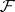of subsets of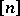that are pairwise unrelated with respect to inclusion is called a Sperner family (or Sperner system) on. Examples are the families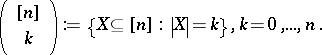Since the binomial coefficients satisfy the inequalities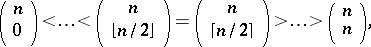in these examples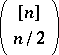, ifis even, andas well as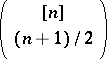, ifis odd, have maximum size. Sperner's theorem from 1928 states that these best examples have even maximum size among all Sperner families onand that they are the only optimal families.

Given a Sperner family, let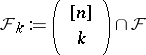and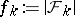. In his original proof, E. Sperner used a shifting technique: Consider the smallestwithand replaceby its upper shadow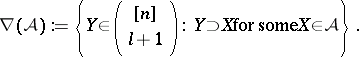Double-counting easily yieldsand, equivalently,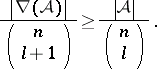(a1)

Thus, each Sperner family can be shifted from below to the "middle" and, analogously, from above to the "middle" and thereby increasing its size.

The inequality (a1) holds for all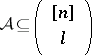and all, and this property is called the normalized matching property of the lattice of subsets of. If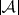andare fixed, the best possible estimate of the upper shadow, and, dually, of the lower shadow (replaceby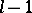and superset by subset), is given by the Kruskal–Katona theorem.

Sperner's theorem follows also easily from the inequalitywhich can be obtained by counting in two different ways the number of pairs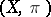where,is a permutation ofand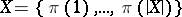. This inequality was proved independently by D. Lubell, S. Yamamoto and L. Meshalkin, and is hence called the LYM inequality; a more general form of it was given by B. Bollobás.

An essential part of Sperner theory consists of the study of other partially ordered sets having analogous properties, e.g. LYM posets and Peck posets (cf. Sperner property).

Details can be found in [a1].